AP Calculus AB : Basic properties of definite integrals (additivity and linearity)

Example Questions

1 3 Next →

Example Question #21 : Basic Properties Of Definite Integrals (Additivity And Linearity)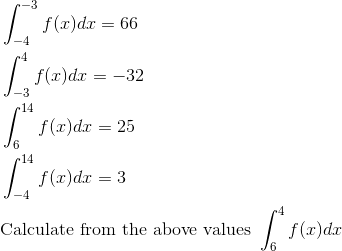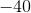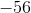Explanation: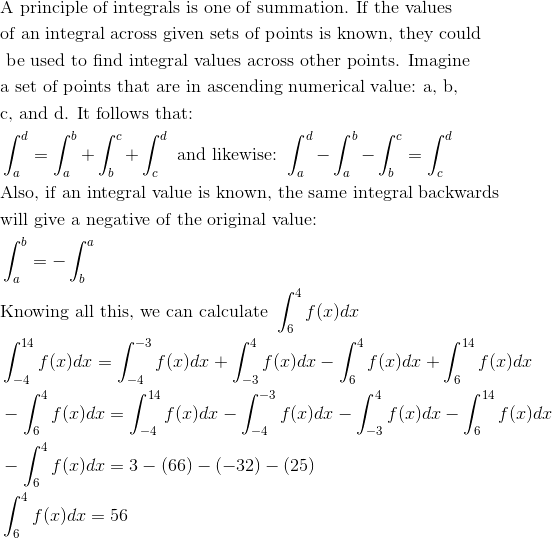Example Question #22 : Basic Properties Of Definite Integrals (Additivity And Linearity)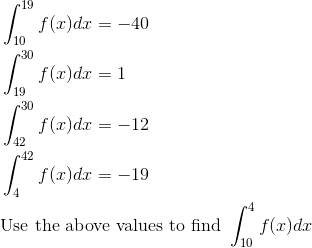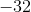Explanation: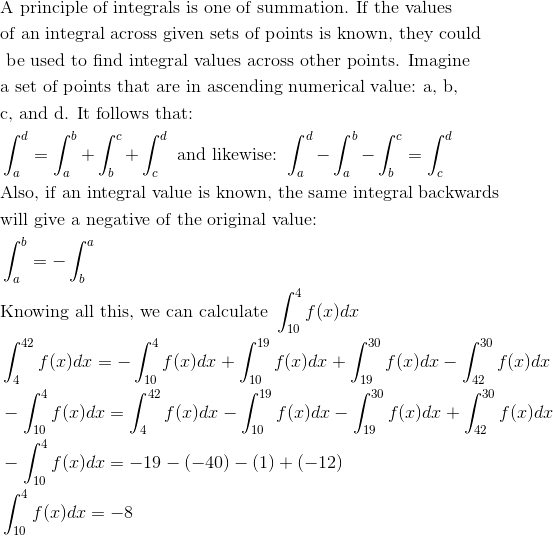Example Question #23 : Basic Properties Of Definite Integrals (Additivity And Linearity)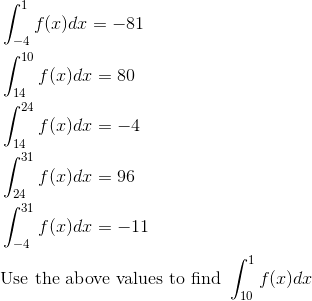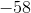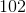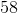Explanation: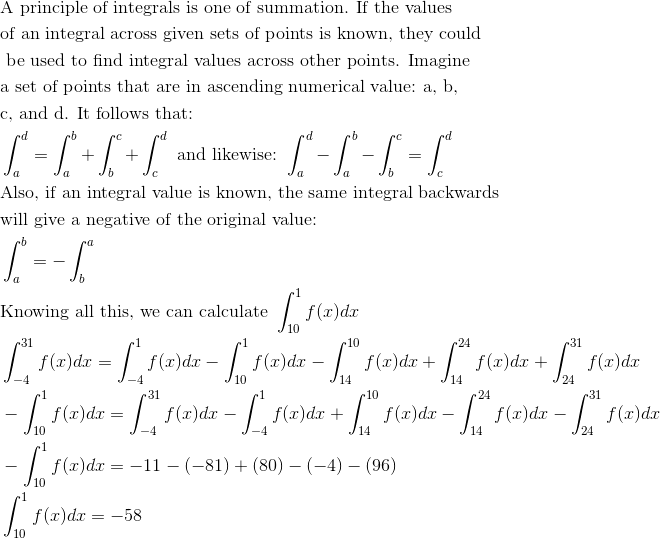Example Question #24 : Basic Properties Of Definite Integrals (Additivity And Linearity)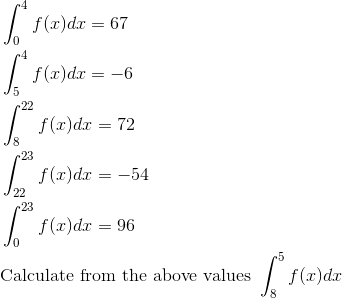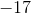Explanation: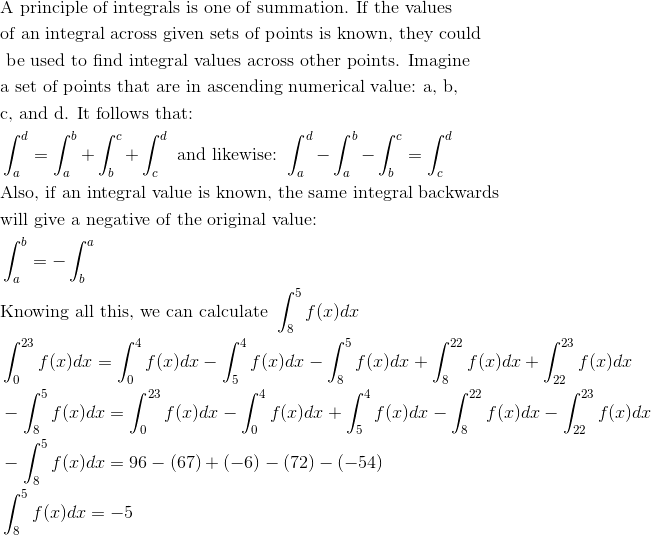Example Question #25 : Basic Properties Of Definite Integrals (Additivity And Linearity)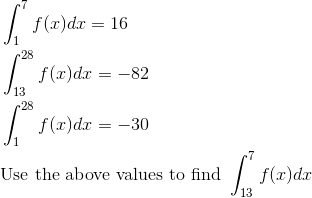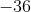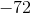Explanation: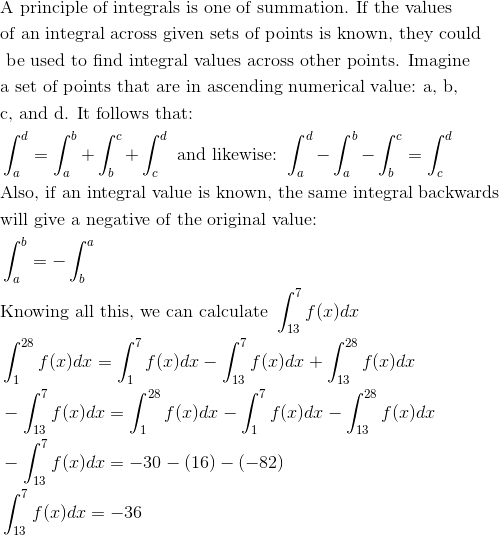Example Question #26 : Basic Properties Of Definite Integrals (Additivity And Linearity)

Given that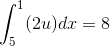and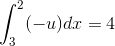, find the value of the following expression: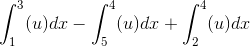Explanation:

First, simplifying the given's gives us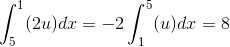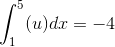And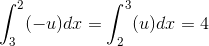Our goal is to get the given expression into terms of these two integrals. Our first step will be to try and get a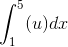from our expression.

First note,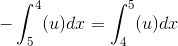And for the third term,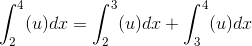Putting these facts together, we can rewrite the original expression as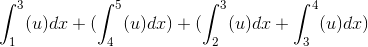Rearranging,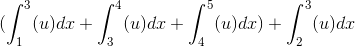The three terms in parentheses can all be brought together, as the top limit of the previous integral matches the bottom limit of the next integral. Thus, we now have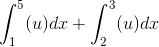Substituting in our given's, this simplifies to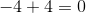Example Question #27 : Basic Properties Of Definite Integrals (Additivity And Linearity)

Evaluate the definite integral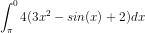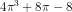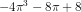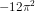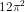Explanation:

Here we are using several basic properties of definite integrals as well as the fundamental theorem of calculus.

First, you can pull coefficients out to the front of integrals.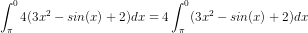Second, we notice that our lower bound is bigger than our upper bound. You can switch the upper and lower bounds if you also switch the sign.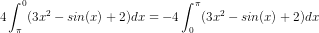Lastly, our integral "distributes" over addition and subtraction. That means you can split the integral by each term and integrate each term separately.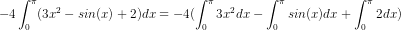Now we integrate and calculate using the Fundamental Theorem of Calculus.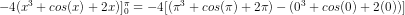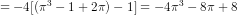Example Question #28 : Basic Properties Of Definite Integrals (Additivity And Linearity)

Solve: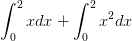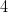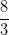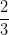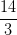Explanation:

Rather than solve each integral independently, we can use the property of linearity to add the integrals together: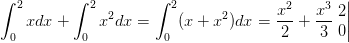The integrals were solved using the following rule: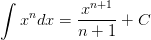Finally, we evaluate the definite integral by evaluating the answer at the upper bound and subtracting the answer evaluated at the lower bound: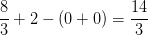1 3 Next →